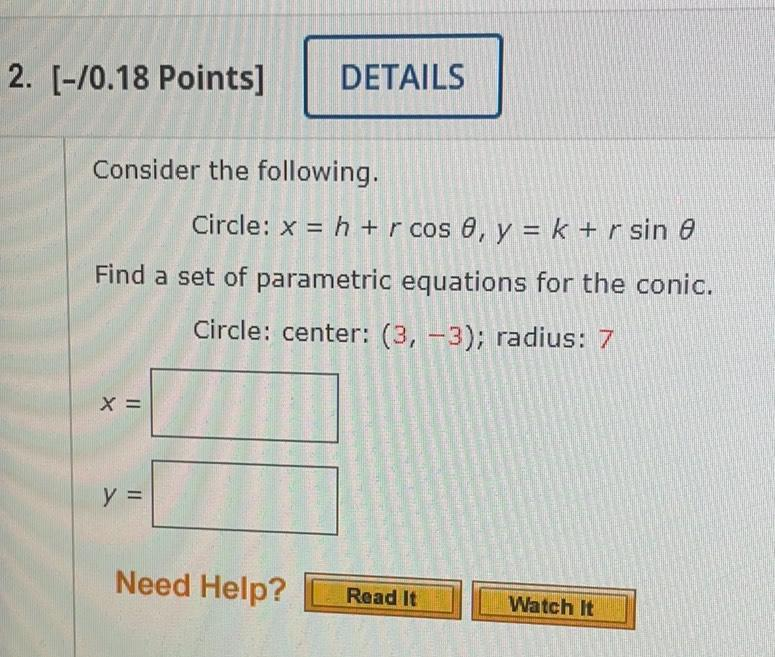Question:

# 2. (-/0.18 Points] DETAILS Consider the following. Circle: x = h + r cos e, y = k + r sin e Find a set of parametric equations f2. (-/0.18 Points] DETAILS Consider the following. Circle: x = h + r cos e, y = k + r sin e Find a set of parametric equations for the conic. Circle: center: (3, -3); radius: 7 X = y = Need Help? Read It Watch It